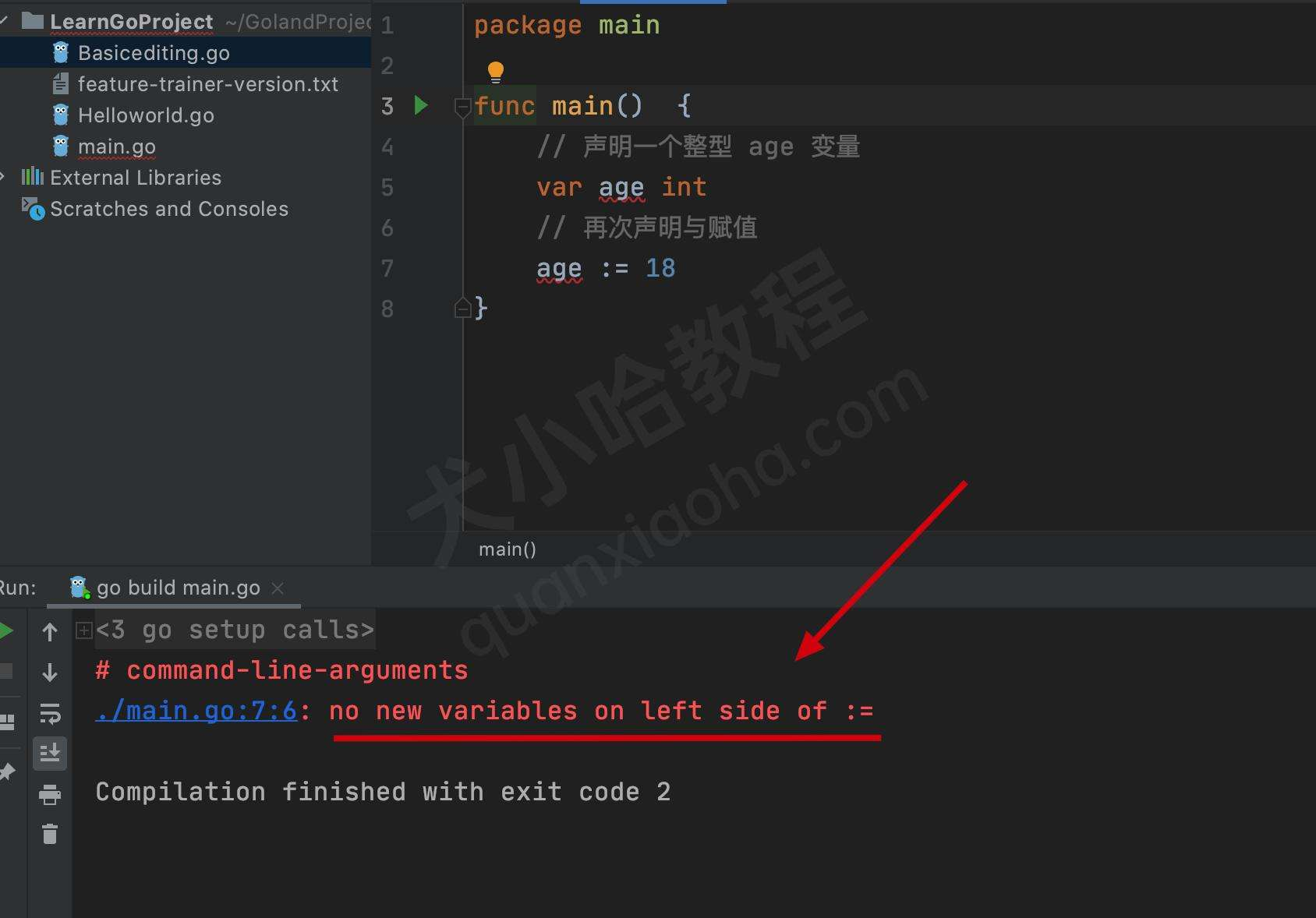# Go 语言变量声明_定义_赋值（图文讲解）

• 整型
• 浮点型
• 布尔型
• 结构体
• ...

Go 语言中不同类型的变量，需要先声明，才能够被使用。

## Go 语言 - 声明变量

### 1. 标准格式

Go 语言中声明变量需以 `var` 关键字开头，变量名放在中间，变量类型放在最后。

``````var 变量名 变量类型
``````

``````var a int                 // 声明一个整型的变量，被用来保存整数数值
var b string              // 声明一个字符串类型的变量
var c []float32           // 声明一个 32 位浮点切片类型的变量
var d func() bool         // 声明一个返回值为布尔型的函数变量，将函数以变量的形式保存起来
var e struct {            // 声明一个结构体类型的变量，结构体中声明了一个整型的 `f` 变量
f int
}
``````

### 2. 批量格式

``````var (
a int
b string
c []float32
d func() bool
e struct {
f int
}
)
``````

## Go 语言 - 初始化与定义变量

Go 语言中，初始化变量有如下几种写法：

### 1. 标准格式

``````var 变量名 变量类型 = 变量值
``````

``````var age int = 18 // 初始化一个整型，变量名为 age, 初始值为 18 的变量
``````

### 2. 编译器推导类型格式

``````var age = 18
``````

### 3. 短变量声明与初始化

``````age := 18
``````

``````// 声明一个整型 age 变量
var age int
// 再次声明与赋值
age := 18
``````

``````no new variables on left side of :=
``````go编译报错

PS: 报错信息意思表示： `:=`左边的变量已经被声明了。

## Go语言 - 多变量同时赋值

``````var a int = 100  // 定义变量 a
var b int = 200  // 定义变量 b
var c int        // 定义一个中间变量

// 开始互相交互 a 与 b 变量的值
c = a
a = b
b = c

fmt.Println(a, b)
``````

``````200 100
``````

``````var a int = 100
var b int = 200

a, b = b, a
fmt.Println(a, b)
``````

``````200 100
``````

## Go 语言 - 匿名变量

• 学生姓名；
• 学生年龄；

``````func findStudent() (string, int) {
return "犬小哈", 100
}

name, _ := findStudent()

fmt.Println(name)
``````

``````犬小哈
``````